# Selina Solutions Concise Mathematics Class 6 Chapter 12: Proportion Exercise 12(B)

Selina Solutions Concise Mathematics Class 6 Chapter 12 Proportion Exercise 12(B), provide students 100% answers relying on mean and continued proportion. Frequent practice of exercise wise problems creates interest among students, which is important from an exam perspective. This also enhances their problem solving skills in Mathematics. Students clear their doubts on the spot, using solutions PDF created by expert teachers. Download Selina Solutions Concise Mathematics Class 6 Chapter 12 Proportion Exercise 12(B) PDF, from the links which are provided below

## Selina Solutions Concise Mathematics Class 6 Chapter 12: Proportion Exercise 12(B) Download PDF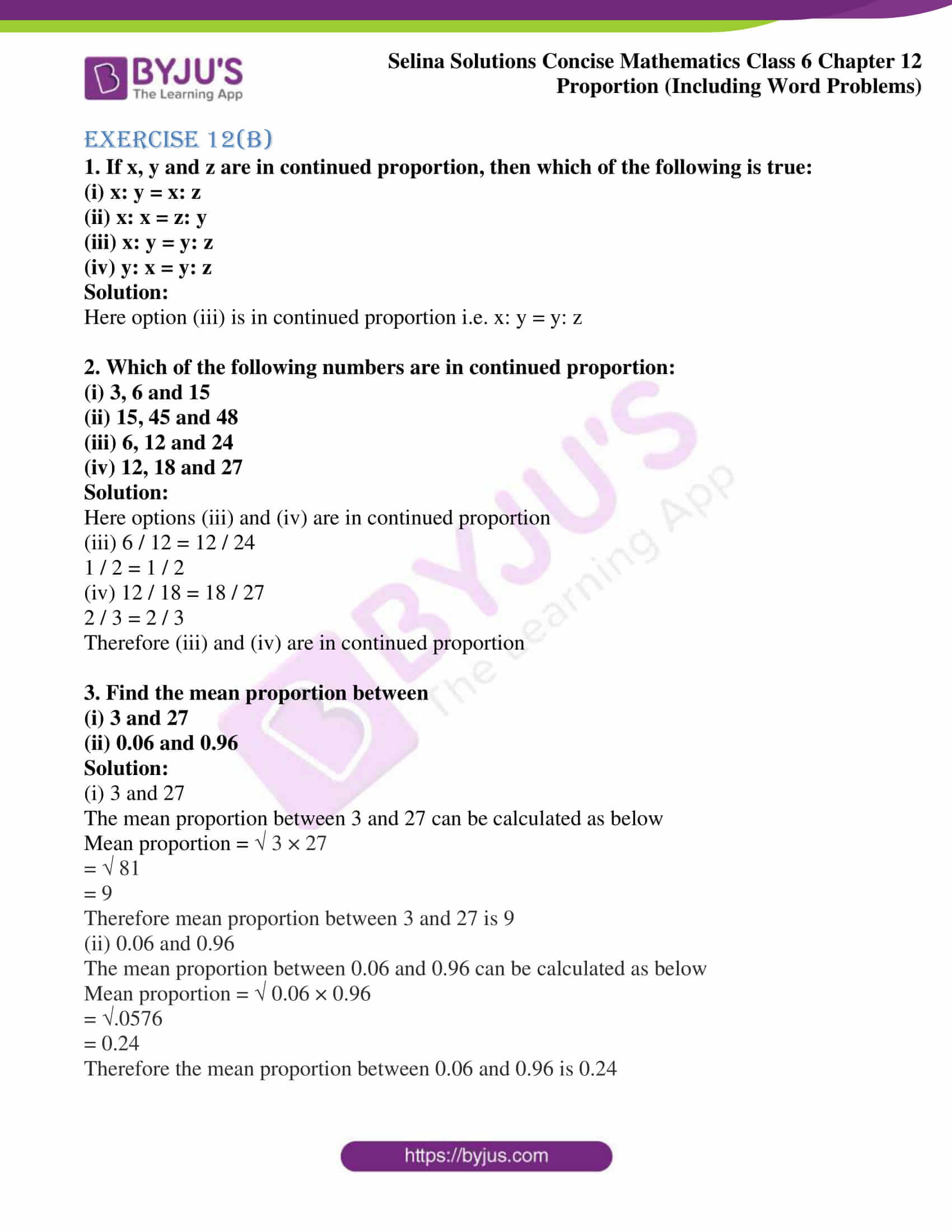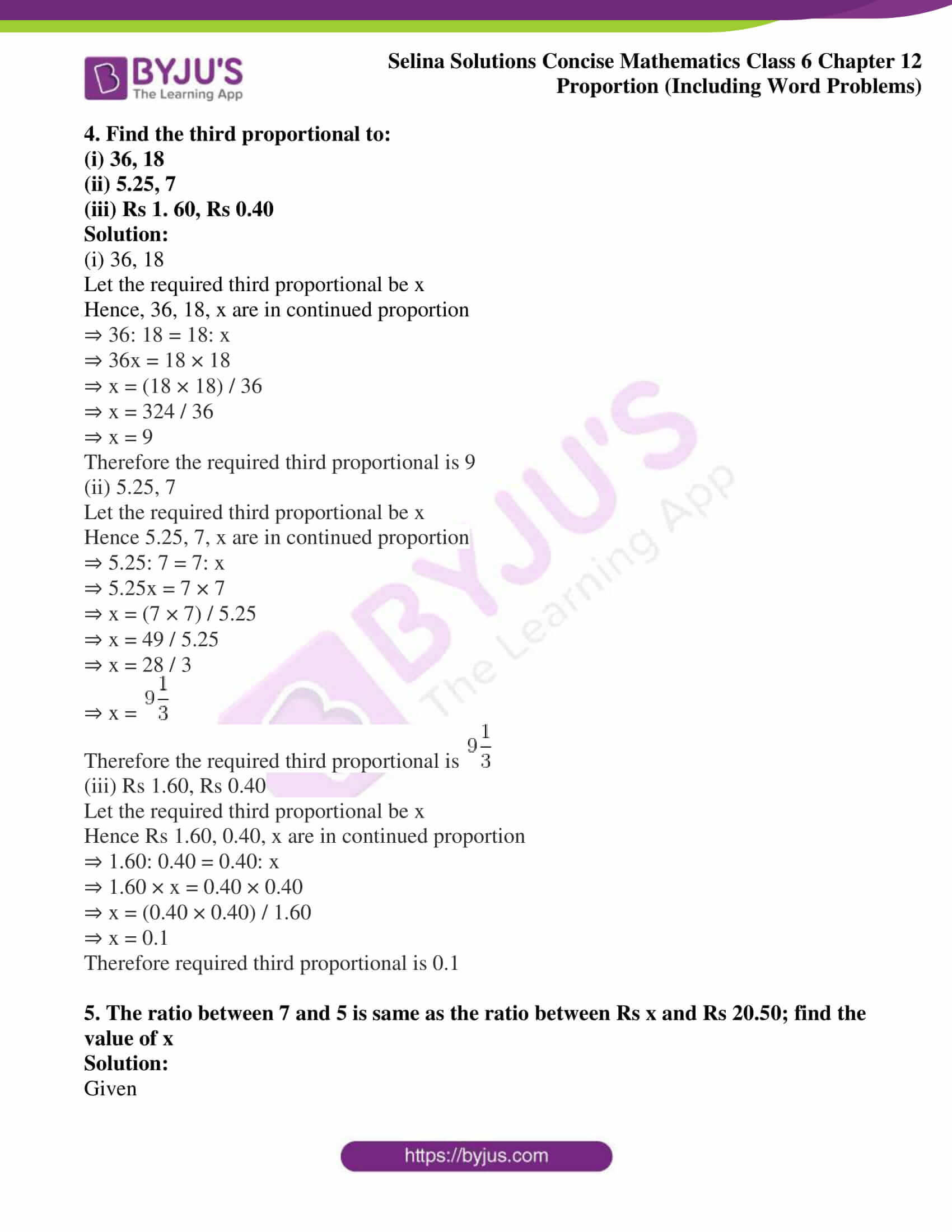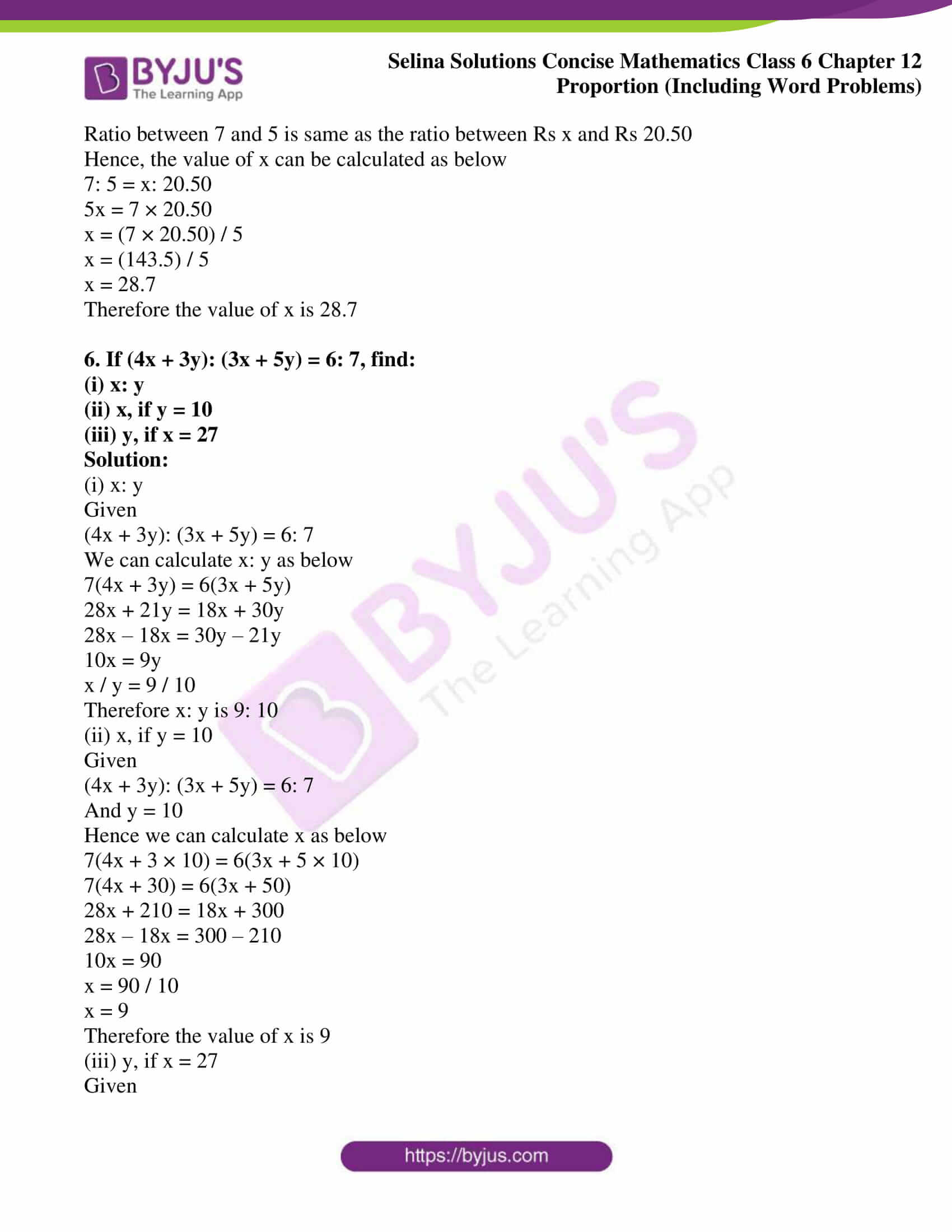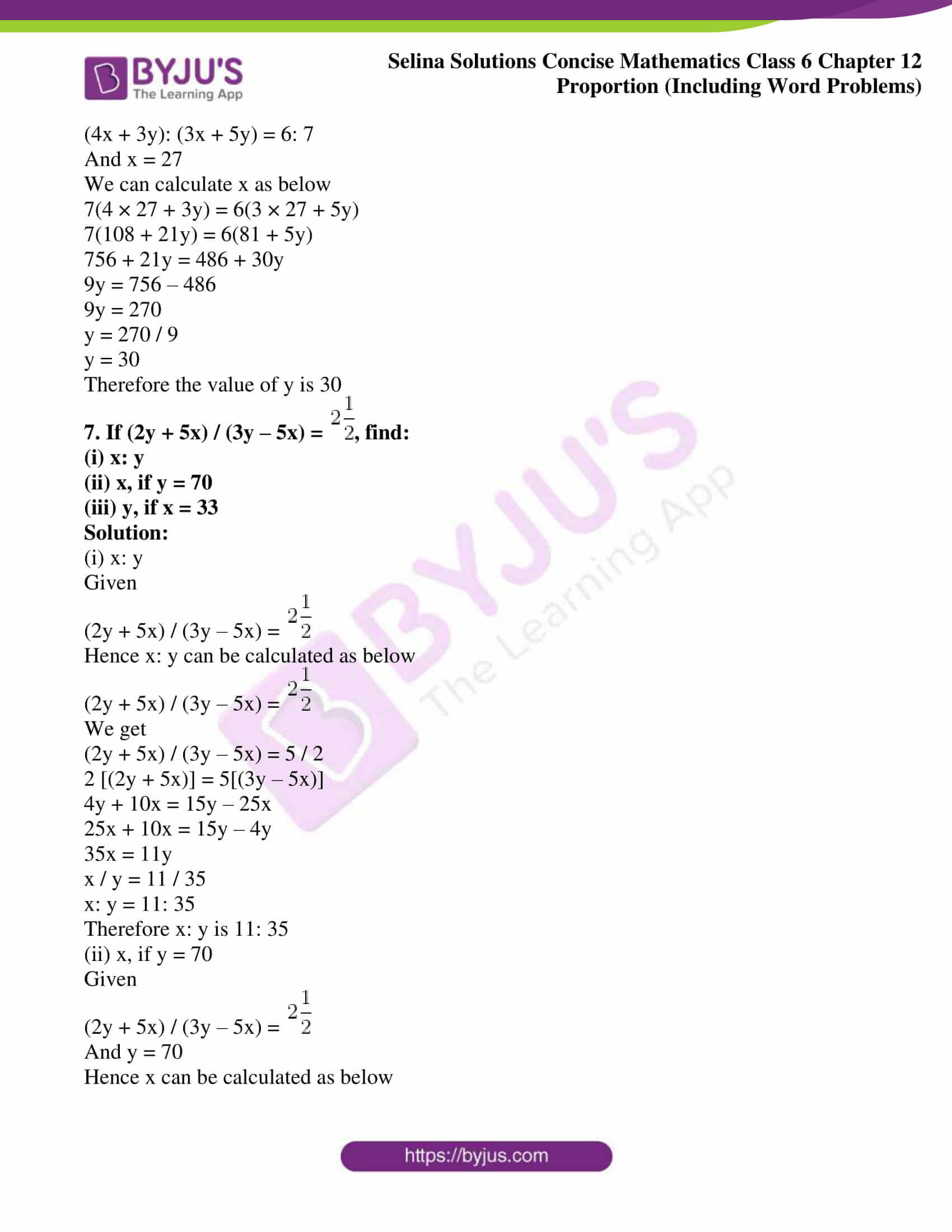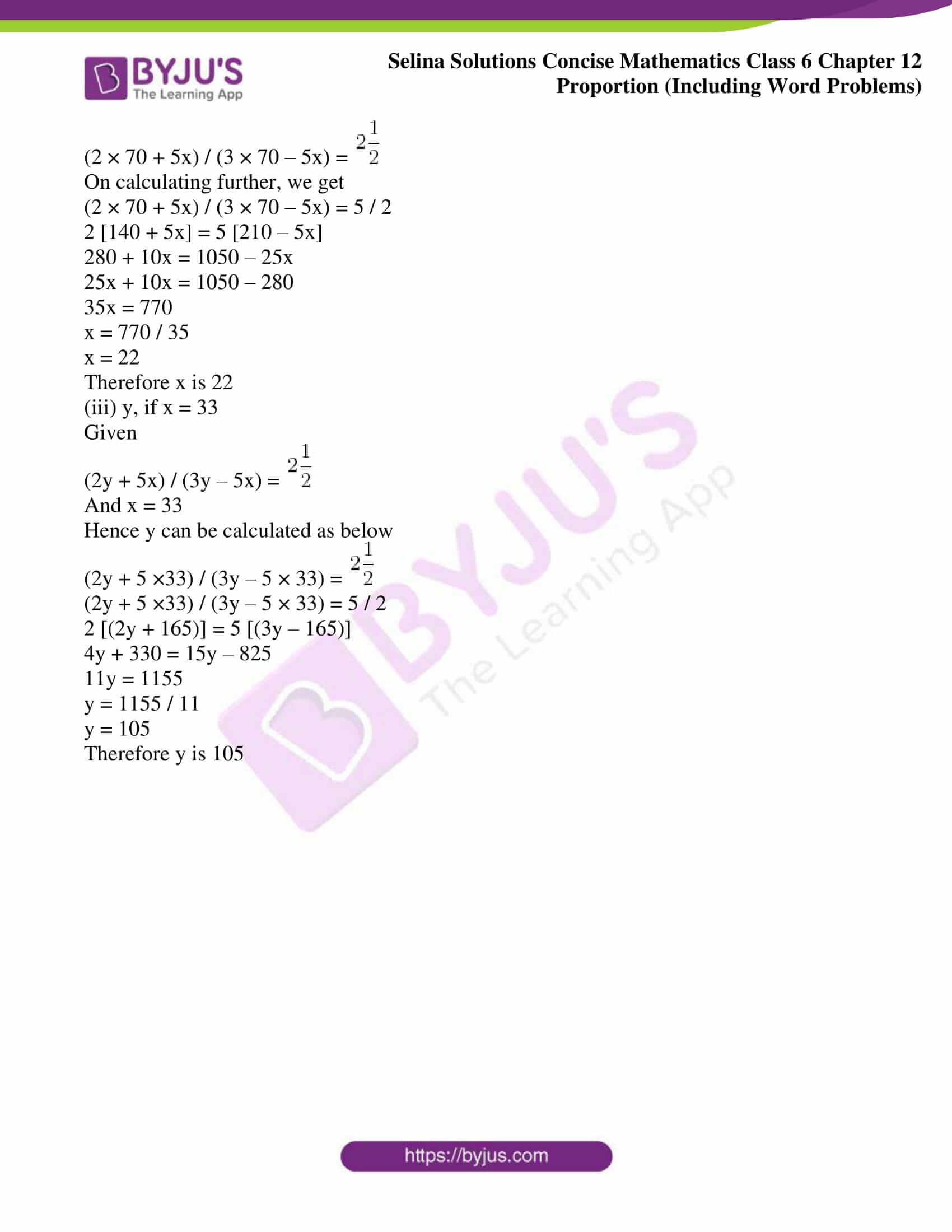### Access Selina Solutions Concise Mathematics Class 6 Chapter 12: Proportion Exercise 12(B)

Exercise 12(B)

1. If x, y and z are in continued proportion, then which of the following is true:

(i) x: y = x: z

(ii) x: x = z: y

(iii) x: y = y: z

(iv) y: x = y: z

Solution:

Here option (iii) is in continued proportion i.e. x: y = y: z

2. Which of the following numbers are in continued proportion:

(i) 3, 6 and 15

(ii) 15, 45 and 48

(iii) 6, 12 and 24

(iv) 12, 18 and 27

Solution:

Here options (iii) and (iv) are in continued proportion

(iii) 6 / 12 = 12 / 24

1 / 2 = 1 / 2

(iv) 12 / 18 = 18 / 27

2 / 3 = 2 / 3

Therefore (iii) and (iv) are in continued proportion

3. Find the mean proportion between

(i) 3 and 27

(ii) 0.06 and 0.96

Solution:

(i) 3 and 27

The mean proportion between 3 and 27 can be calculated as below

Mean proportion = 3 × 27

=  81

= 9

Therefore mean proportion between 3 and 27 is 9

(ii) 0.06 and 0.96

The mean proportion between 0.06 and 0.96 can be calculated as below

Mean proportion =  0.06 × 0.96

= .0576

= 0.24

Therefore the mean proportion between 0.06 and 0.96 is 0.24

4. Find the third proportional to:

(i) 36, 18

(ii) 5.25, 7

(iii) Rs 1. 60, Rs 0.40

Solution:

(i) 36, 18

Let the required third proportional be x

Hence, 36, 18, x are in continued proportion

⇒ 36: 18 = 18: x

⇒ 36x = 18 × 18

⇒ x = (18 × 18) / 36

⇒ x = 324 / 36

⇒ x = 9

Therefore the required third proportional is 9

(ii) 5.25, 7

Let the required third proportional be x

Hence 5.25, 7, x are in continued proportion

⇒ 5.25: 7 = 7: x

⇒ 5.25x = 7 × 7

⇒ x = (7 × 7) / 5.25

⇒ x = 49 / 5.25

⇒ x = 28 / 3

⇒ x =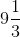Therefore the required third proportional is(iii) Rs 1.60, Rs 0.40

Let the required third proportional be x

Hence Rs 1.60, 0.40, x are in continued proportion

⇒ 1.60: 0.40 = 0.40: x

⇒ 1.60 × x = 0.40 × 0.40

⇒ x = (0.40 × 0.40) / 1.60

⇒ x = 0.1

Therefore required third proportional is 0.1

5. The ratio between 7 and 5 is same as the ratio between Rs x and Rs 20.50; find the value of x

Solution:

Given

Ratio between 7 and 5 is same as the ratio between Rs x and Rs 20.50

Hence, the value of x can be calculated as below

7: 5 = x: 20.50

5x = 7 × 20.50

x = (7 × 20.50) / 5

x = (143.5) / 5

x = 28.7

Therefore the value of x is 28.7

6. If (4x + 3y): (3x + 5y) = 6: 7, find:

(i) x: y

(ii) x, if y = 10

(iii) y, if x = 27

Solution:

(i) x: y

Given

(4x + 3y): (3x + 5y) = 6: 7

We can calculate x: y as below

7(4x + 3y) = 6(3x + 5y)

28x + 21y = 18x + 30y

28x – 18x = 30y – 21y

10x = 9y

x / y = 9 / 10

Therefore x: y is 9: 10

(ii) x, if y = 10

Given

(4x + 3y): (3x + 5y) = 6: 7

And y = 10

Hence we can calculate x as below

7(4x + 3 × 10) = 6(3x + 5 × 10)

7(4x + 30) = 6(3x + 50)

28x + 210 = 18x + 300

28x – 18x = 300 – 210

10x = 90

x = 90 / 10

x = 9

Therefore the value of x is 9

(iii) y, if x = 27

Given

(4x + 3y): (3x + 5y) = 6: 7

And x = 27

We can calculate x as below

7(4 × 27 + 3y) = 6(3 × 27 + 5y)

7(108 + 21y) = 6(81 + 5y)

756 + 21y = 486 + 30y

9y = 756 – 486

9y = 270

y = 270 / 9

y = 30

Therefore the value of y is 30

7. If (2y + 5x) / (3y – 5x) =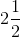, find:

(i) x: y

(ii) x, if y = 70

(iii) y, if x = 33

Solution:

(i) x: y

Given

(2y + 5x) / (3y – 5x) =Hence x: y can be calculated as below

(2y + 5x) / (3y – 5x) =We get

(2y + 5x) / (3y – 5x) = 5 / 2

2 [(2y + 5x)] = 5[(3y – 5x)]

4y + 10x = 15y – 25x

25x + 10x = 15y – 4y

35x = 11y

x / y = 11 / 35

x: y = 11: 35

Therefore x: y is 11: 35

(ii) x, if y = 70

Given

(2y + 5x) / (3y – 5x) =And y = 70

Hence x can be calculated as below

(2 × 70 + 5x) / (3 × 70 – 5x) =On calculating further, we get

(2 × 70 + 5x) / (3 × 70 – 5x) = 5 / 2

2 [140 + 5x] = 5 [210 – 5x]

280 + 10x = 1050 – 25x

25x + 10x = 1050 – 280

35x = 770

x = 770 / 35

x = 22

Therefore x is 22

(iii) y, if x = 33

Given

(2y + 5x) / (3y – 5x) =And x = 33

Hence y can be calculated as below

(2y + 5 ×33) / (3y – 5 × 33) =(2y + 5 ×33) / (3y – 5 × 33) = 5 / 2

2 [(2y + 165)] = 5 [(3y – 165)]

4y + 330 = 15y – 825

11y = 1155

y = 1155 / 11

y = 105

Therefore y is 105